# Math in Focus Grade 5 Cumulative Review Chapters 1 and 2 Answer Key

Go through the Math in Focus Grade 5 Workbook Answer Key Cumulative Review Chapters 1 and 2 to finish your assignments.

## Math in Focus Grade 5 Cumulative Review Chapters 1 and 2 Answer Key

Concepts and Skills

Write each number in standard form. (Lesson 1.1)

Question 1.
One hundred thousand, seventy _____
100,070,

Explanation:
Standard form or expanded notation is a way of writing numbers to see the math value of individual digits.
Numbers are separated into individual place values to write in numbers.
One hundred thousand = 100 x 1000,
Seventy = 7 x 10,
Add both 100,000 + 70 = 100,070.

Question 2.
Five hundred sixty thousand _____
560,000,

Explanation:
Standard form or expanded notation is a way of writing numbers to see the
math value of individual digits.
Numbers are separated into individual place values to write in numbers.
Five hundred sixty thousand = 560 x 1000 = 560,0 00.

Question 3.
Five million, eighty thousand, five _____
50,080,005,

Explanation:
Standard form or expanded notation is a way of writing numbers to see the
math value of individual digits.
Numbers are separated into individual place values to write in numbers.
Five million = 5 x 1,000,000,
Eighty thousand = 80 x 1000,
Five = 5,
Add all the numbers = 50,080,005.

Question 4.
Two million, four hundred thousand, seven hundred twenty ___
2,400,720,

Explanation:
Standard form or expanded notation is a way of writing numbers to see the
math value of individual digits.
Numbers are separated into individual place values to write in numbers.
Two million = 2 x 1,000,000,
Four hundred thousand = 400 x 1000,
Seven hundred twenty = 720,

Write each number in word form. (Lesson 1.1)

Question 5.
120, 450 _____
One hundred twenty thousand four hundred fifty,

Explanation:
Word form  and stand forms are both are reciprocal to each other.
Numbers are separated into individual place values to write in word form.

Question 6.
500, 312 _______
Five hundred thousand three hundred twelve,

Explanation:
Word form  and stand forms are both are reciprocal to each other.
Numbers are separated into individual place values to write in word form.

Question 7.
1,050,400 _____
One million fifty thousand four hundred,

Explanation:
Word form  and stand forms are both are reciprocal to each other.
Numbers are separated into individual place values to write in word form.

Question 8.
5,732,800 _____
Five million seven hundred thirty-two thousand eight hundred,

Explanation:
Word form  and stand forms are both are reciprocal to each other.
Numbers are separated into individual place values to write in word form.

Complete. (Lesson 1.2)

In 1,238,906:
One million two hundred thirty-eight thousand nine hundred six

Question 9.
the digit 8 stands for _____
8 stands for eight thousand,

Explanation:
Count the place values from right hand side at first,
So, 8 stands in thousand’s place.

Question 10.
the digit 9 stands for _____
9 stands for nine hundred,

Explanation:
Count the place values from right hand side at first,
So, 9 stands in hundred’s place.

Question 11.
the digit 1 stands for _____
1 stands for one million,

Explanation:
Count the place values from right hand side at first,
So, 1 stands in million’s place.

State the value of the digit 3 in each number. (Lesson 1.2)

Question 12.
538,426: ____
The value of the digit 3 is thirty thousand,

Explanation:
Count the place values from right hand side at first,
So, 3 stands in thousand’s place.

Question 13.
1,325,407: ____
The value of the digit 3 is three hundred thousands,

Explanation:
Count the place values from right hand side at first.
So, 3 stands in 300 thousand’s place.

Complete. (Lesson 1.2)

Question 14.
In 807,456, the digit ____ is in the thousands place.
7 is in the thousands place,

Explanation:
Count the place values from right hand side to the know the place value of 7,
So, 7 is in thousand’s place.

Question 15.
In 5,486,302, the digit ___ is in the millions place.
5 is in the millions place,

Explanation:
Count the place values from right hand side to know the place value of 5,
So, 5 is in millions place.

Question 16.
In 305,128, the digit 0 is in the ____ place.
0 is in the ten thousand place,

Explanation:
Count the place values from right hand side to know the place value of 0,
So, 0 is in ten thousand’s place.

Question 17.
In 7,614,892, the digit 6 is in the ____ place.
6 is in the hundred thousand’s place,

Explanation:
Count the place values from right hand side to know the place value of 6,
So, 6 is in hundred thousand’s place.

Question 18
918,230 = ___ + 10,000 + 8,000 + 200 + 30
9,00,000,

Explanation:
Count the place values from right hand side to know the place value of 9.
So, 9 is in millions’ place.
918,230 = 900,000 + 10,000 + 8,000 + 200 + 30.

Question 19.
538,417 = 500,000 + ____ + 8,000 + 400 + 10 + 7
30,000,

Explanation:
Count the place values from right hand side to know the place value of 9.
So, 3 is in ten thousand’s place.
538,417 = 500,000 + 30000 + 8,000 + 400 + 10 + 7.

Question 20.
6,000,000 + 30,000 + 90 = _____
9,030,090,

Explanation:
Standard form or expanded notation is a way of writing numbers to see the
math value of individual digits,
6,000,000 + 30,000 + 90 =9,030,090.

Fill each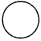with >or <‘. (Lesson 1.3)

Question 21.
185,263183,256
185,263 > 183,256,

Explanation:
First compare both the numbers,
by subtracting the smaller number 183,256 from bigger number 185,263,
then put the appropriate symbol in the circle.

Question 22.
5,060,345995,863
5,060,345 > 995,863,

Explanation:
Count the place values of both the numbers.
in 5,060,345 place values are more.
So, put the greater than symbol in the circle.

Question 23.
899,506900,650
899,506 < 900,650,

Explanation:
First compare both the numbers,
by subtracting the smaller number 899,506 from bigger number 900,650
then put the appropriate symbol in the circle.

Question 24.
231623231,621
231,623 > 231,621,

Explanation:
First compare both the numbers,
by subtracting the smaller number 231,621 from bigger number 231,621
then put the appropriate symbol in the circle.

Order the number from greatest to least. (Lesson 1.3)

Question 5.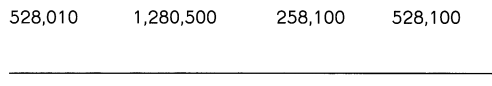1,280,500    528,100    5280,100   528,010,

Explanation:
Arranging the numbers from greatest to smallest is known as descending order.

Find the rule. Then complete the number pattern. (Lesson 1.3)

Question 26.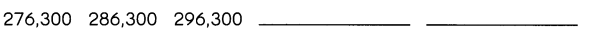Rule: ________
276,300   286,300    296,300    306,300    316,300,

Rule:
n + 1000,

Explanation:
Number patterns are the patterns in which a list of numbers follows a certain sequence.
Generally, the patterns establish the relationship between two numbers.
It is also known as the sequences of series in numbers.

Estimate by rounding. (Lesson 1.4)

Question 27.
7,512 + 3,281 _______
10,800,

Explanation:
Estimate of 7,512 is 7,500,
Estimate of 3,281 is 3,300,
7,500 + 3,300 = 10,800.

Question 28.
6,528 – 5,938 _______
500,

Explanation:
Estimate of 6,528 is 6500,
Estimate of 5,938 is 6000,
6,500 – 6,000  = 500.

Question 29.
1,592 × 5 _____
80,000,

Explanation:
Estimate of 1,592 is 1600,
1,600 x 5 = 8,000.

Question 30.
2,576 ÷ 3 _______
866,

Explanation:
Estimate of 2,576 is 2,600,
2,600 ÷ 3 =  866.

Estimate using front-end estimation with adjustment. (Lesson 1.4)

Question 31.
4,087 + 3,910 + 9,125
17,000,

Explanation:
Estimate of 4,087 is 4,000,
Estimate of 3,910 is 4,000,
Estimate of 9,125 is 9,000,
4,000 + 3,000 + 9,000 = 16,000,
000 + 900 + 100 = 1,000,
16,000 + 1,000 = 17,000.

Estimate using front-end estimation with adjustment. (Lesson 1.4)

Question 32.
8,685 + 6,319 + 7,752
22,700,

Explanation:
Estimate of 8,685 is 8,000,
Estimate of 6,319 is 6,000,
Estimate of 7,752 is 7,000,
8,000 + 6,000 + 7,000 = 21,000,
600 + 300 + 800 = 1,700,
21,000 + 1,700 = 22,700.

Question 33.
5,879 – 1,143
4,800,

Explanation:
Estimate of 5,879 is 5,000,
Estimate of 1,143 is 1,000,
5,000 – 1,000 = 4,000,
900 – 100 = 800,
4,000 + 800 = 4,800.

Question 34.
7,974 – 2,660
5,200,

Explanation:
Estimate of 7,974 is 7,000,
Estimate of 2,660 is 2,000,
7,000 – 2,000 = 5,000,
900 – 700 = 200,
5,000 + 200 = 5,200.

You may use your calculator where necessary. (Lesson 2.1)

Question 35.
Find the area of a square that has sides of length 96 inches.
________
9,216 square inches,

Explanation:
Area of a square = side x side,
Area = 96 x 96 square inches,
Area = 9,216 square inches.

Question 36.
Ms. Suarez has $5,651. Mr. Knox, has$853 more than Ms. Suarez. How much does Mr. Knox have?
___________
$6504, Explanation: Ms. Suarez has$5,651,
Mr. Knox has $5,651 +$853 = $6504, Mr. Knox have$6504.

You may use your calculator where necessary. (Lesson 2.1)

Question 37.
There are 176 gallons of gas in Tank A. There are 19 gallons less gas in Tank B.
How many gallons of gas are there in Tank B?
__157 gallons of gas______
157 gallons of gas,

Explanation:
Tank A    176 gallons of gas,
Tank B    176 – 19 = 157 gallons of gas.

Question 38.
A truck is loaded with 25 similar crates. The total weight of the crates is 2,000 pounds.
What is the weight of each crate?
___80 pounds______
80 pounds,

Explanation:
A truck is loaded with 25 similar crates,
The total weight of the crates is 2,000 pounds,
The weight of each crate 2,000 ÷ 25 = 80 pounds.

Multiply. (Lesson 2.2)

Question 39.
315 × 10 = __3,150_____
3,150,

Explanation:
315 x 10 = 300 x 10 + 10 x 10 + 5 x 10,
= 3000 + 100 + 50,
= 3,150.

Question 40.
25 × 100 = _2,500___
2,500,

Explanation:
100 x 25 = 20 x 100 + 5 x 100,
= 2000 + 500,
= 2,500.

Question 41.
238 × 1,000 = __238,000__
238,000,

Explanation:
238 x 1000 = 200 x 1000 + 30 x 1000 + 8 x 1000,
= 2,00,000 + 30,000 + 8,000,
= 238,000.

Question 42.
147 × 50 = _7,350___
7,350,

Explanation:
147 x 50 = 100 x 50 + 40 x 50 + 7 x 50,
= 5,000 + 2,000 + 350,
= 7,350.

Question 43.
63 × 200 = _12,600___
12,600,

Explanation:
60 x 200 + 3 x 200 = 12000 + 600,
= 12,600.

Question 44.
906 × 7,000 = __6,342,000__
6,342,000,

Explanation:
906 x 7,000 = 900 x 7,000 + 0 x 6,000 + 6 x 7,000,
=6,300,000 + 0 + 42,000
= 6,342,000.

Estimate by rounding. (Lesson 2.2)

Question 45.
41 × 58 ______
2,400,

Explanation:
41 x 58 = 40 x 60,
= 2,400.

Question 46.
297 × 32 _____
9,000,

Explanation:
300 x 30 = 9,000.

Question 47.
1,087 × 21= __22,000___
22,000,

Explanation:
1,100 x 20 = 22,000.

Question 48.
4.975 × 78 = __388.05___
388.05,
Estimate: 400,

Explanation:
4.975 X 78 =388.05,
Estimate of 4.975 is 5,
Estimate of 78 is 80,
So, 5 x 80 = 400.

Multiply. (Lesson 2.3)

Question 49.
19 × 102 = ____
1,900,

Explanation:
19 x 102,
102 = 10 x 10 = 100,
= 19 x 100,
= 1,900.

Question 50.
186 × 102 = __18,600__
18,600,

Explanation:
186 x 102 = 102 = 10 x 10 = 100,
= 186 x 100,
= 100 x 100 + 80 x 100 + 6 x 100,
= 10,000 + 8,000 + 600,
= 18,600.

Question 51.
65 × 103 = __65,000__
65,000,

Explanation:
65 x 103,
103 = 10 x 10 x 10 = 1,000,
= 65 x 1,000,
= 65,000.

Question 52.
154 × 103 = _1,54,000__
1,54,000,

Explanation:
154 x 103 = 103 = 10 x 10 x 10 = 1,000,
=154 x 1,000,
= 1,54,000.

Question 53.
82 × 45 = ____
3,600,

Explanation:
Estimate of 82 is 80, 80 x 45 = 80 x 40 + 80 x 5,
= 3,200 + 400,
= 3,600,

Check
82 x 45,
Multiply 82 by 5,
Multiply 82 by 4 tens,
410 + 3,280 = 3,690,

Question 54.
78 × 21 = __1,600___
1,600,

Explanation:
Given 78 X 21 we
Estimate of 78 is 80,80 x 20 = 1,600,
Check
78 x 21,
Multiply 78 by 20,
Multiply 78 by 1 ten,
1,560 + 78 = 1,638,

Question 55.
275 × 59 = __16,500__
16,500,

Explanation:
275 x 60 = 200 x 60 + 70 x 60 + 5 x 60,
= 12000 + 4200 + 300,
= 16,500
Check
275 x 59 = 16,225.

Question 56.
738 × 96 = _74,000___
74,000,

Explanation:
= 740 x 100 = 700 x 100 + 40 x 100 + 0 x 100,
= 70,000 + 4,000 + 0,
= 74,000,
Check
738 x 96 = 70,848,

Question 57.
4,672 × 73 = _327,040___
4,672 x 73,
4,672 x 70 = 327,040,
4,672 x 3 = 14,016,
327,040+14,016 = 341,056,
Check
4,700 x 70 = 3,29,000,

Question 58.
8,781 × 26 = _175,620___
8,672, x 20 = 175,620,
8,781 x 6 = 52,686,
175,620 + 52,686 = 2,28,306,
8,800 x 25 = 2,20,000.

Divide. (Lesson 2.5)

Question 59.
3,560 ÷ 10 = _356__
356,

Explanation:
3,560 ÷ 10,
3,560 is dividend and 10 is divisor
Count the number of zeros in dividend and divisor and
just move the decimal point one point to the left side.

Question 60.
1,900 ÷ 100 = _19___
19,

Explanation:
1,900 ÷ 100,
1,900 is dividend and 10 is divisor
Count the number of zeros in dividend and divisor and
just move the decimal point two points to the left side.

Question 61.
17,000 ÷ 1,000 = __17__
17,

Explanation:
17,000 ÷ 1,000,
17,000 is dividend and 1,000 is divisor
Count the number of zeros in dividend and divisor and
just move the decimal point 3 points to the left side.

Question 62.
900 ÷ 60 = __15__
15,

Explanation:
900 ÷ 60 = (900 ÷ 10) ÷ 6,
= 90 ÷ 6 =  15.

Question 63.
96,000 ÷ 400 = __240__
240,

Explanation:
96,00 ÷ 400 = (96,000 ÷ 100) ÷ 4,
= 9,60 ÷ 4,
=  240.

Question 64.
504,000 ÷ 9,000 = _56__
56,

Explanation:
504,000 ÷ 9,000 = (504,000 ÷ 1000) ÷ 9,
= 504 ÷ 9,
=  56.

Estimate. (Lesson 2.5)

Question 65.
4,593 ÷ 53 = __90__
90,

Explanation:
Estimate of 4,593 is 4,500,
Estimate of 53 is 50,
So, 4500 ÷ 50 = 90,
50 x 90 = 4,500.

Question 66.
6,298 ÷ 164 = __30__
30,

Explanation:
Estimate of 6,298 is 6,000,
Estimate of 164 is 200,
So, 6,000 ÷ 200 = 30,
200 x 30 = 6,000.

Question 67.
7,623 ÷ 4,451 = __2__
2,

Explanation:
Estimate of 7,623 is 8,000,
Estimate of 4,451 is 4,000,
So, 8,000 ÷ 4,000 = 2,
4,000 x 2 = 8,000.

Question 68.
4,239 ÷ 73 = _60___
60,

Explanation:
Estimate of 4,239 is 4,200,
Estimate of 73 is 70,
So, 4200 ÷ 70 = 60,
70 x 60 = 4,200,

Divide. (Lesson 2.6)

Question 69.
96 ÷ 16 = _6__
6,

Explanation:
96 ÷ 16,
16 rounds to 10.
6 × 10 = 60,
6 × 16 = 96,

Question 70.
57 ÷ 23 = __2 R 11__
2 R 11,

Explanation:
57 ÷ 23,
23 rounds to 20.
3 × 20 = 60,
3 × 23 = 69,
The estimated quotient is too big. Try 2.

Question 71.
459 ÷ 27 = _17___
17,

Explanation:
459 ÷ 27,
459 rounds to 500.
27 rounds to 30,
500 ÷ 30 = 16.6,

Question 72.
503 ÷ 15 = _33 R 8___
33 R 8,

Explanation:
503 ÷ 15,
503 rounds to 500.
15 rounds to 20,
20 x 25 = 500,
The quotient is about 33 and remainder is 8.

Simplify. (Lesson 2.7)

Question 73.
60 + 12 – 36 = _36__
36,

Explanation:
The order of operation in math is a set of rules revolving around 4 major operators.
According to the order of operations, there is a particular sequence which we need to follow,
on each operator while solving the given mathematical expression we apply DMAS rule.
DMAS is the elementary rule for the order of operation of the Binary operations.
This States that Division will be done before Multiplication,
Step 1: 60 + 12 = 72,
Step 2: 72 – 36 = 36.

Question 74.
10 × 9 ÷ 3 = __30__
30,

Explanation:
The order of operation in math is a set of rules revolving around 4 major operators.
According to the order of operations, there is a particular sequence which we need to follow,
on each operator while solving the given mathematical expression we apply DMAS rule.
DMAS is the elementary rule for the order of operation of the Binary operations.
This States that Division will be done before Multiplication,
Step 1: 9 ÷ 3 = 3,
Step 2: 10 x 3 = 30.

Question 75.
29 + 42 ÷ 6 = _36__
36,

Explanation:
The order of operation in math is a set of rules revolving around 4 major operators.
According to the order of operations, there is a particular sequence which we need to follow,
on each operator while solving the given mathematical expression we apply DMAS rule.
DMAS is the elementary rule for the order of operation of the Binary operations.
This States that Division will be done before Multiplication,
Step 1: 42 ÷ 6 = 7,
Step 2: 29 + 7 = 36.

Question 76.
(90 – 85) × 7 = _35__
35,

Explanation:
The order of operation in math is a set of rules revolving around 4 major operators.
According to the order of operations, there is a particular sequence which we need to follow,
on each operator while solving the given mathematical expression we apply DMAS rule.
DMAS is the elementary rule for the order of operation of the Binary operations.
This States that Division will be done before Multiplication,
Step 1 : 90 – 85 = 5,
Step 2: 5 x 7 = 35.

Question 77.
50 × 8 + 12 ÷ 4 = _403___
403,

Explanation:
The order of operation in math is a set of rules revolving around 4 major operators.
According to the order of operations, there is a particular sequence which we need to follow,
on each operator while solving the given mathematical expression we apply DMAS rule.
DMAS is the elementary rule for the order of operation of the Binary operations.
This States that Division will be done before Multiplication,
Step 1: 12 ÷ 4 = 3,
Step 2: 50 x 8 = 400,
Step 3: 400 + 3 = 403.

Question 78.
69 ÷ 3 – 3 + 10 = _30__
30,

Explanation:
The order of operation in math is a set of rules revolving around 4 major operators.
According to the order of operations, there is a particular sequence which we need to follow,
on each operator while solving the given mathematical expression we apply DMAS rule.
DMAS is the elementary rule for the order of operation of the Binary operations.
This States that Division will be done before Multiplication,
Step 1: 69 ÷ 3 = 23
Step 2: 23 – 3 = 20
Step 3: 20 + 10 = 30.

Evaluate. (Lesson 2.7)

Question 79.
56 + {12 – [18 – (3 + 9)]} = _62___
62,

Explanation:
According to the order of operations, there is a particular sequence which we need to follow,
on each operator while solving the given mathematical expression we apply BODMAS rule.
BODMAS is the elementary rule for the order of operation of the Binary operations.
The acronym stands for B – Brackets, O – Order of powers, D – Division,
M – Multiplication, A – Addition, and S – Subtraction.
Step 1: 3 + 9 = 12,
Step 2: 18 – 12 = 6,
Step 3: 12 – 6 = 6,
Step 4: 56 + 6 = 62.

Question 80.
100 ÷ (20 + 5) + [(18 – 3) × 4] = __64__
64,

Explanation:
According to the order of operations, there is a particular sequence which we need to follow,
on each operator while solving the given mathematical expression we apply BODMAS rule.
BODMAS is the elementary rule for the order of operation of the Binary operations.
The acronym stands for B – Brackets, O – Order of powers, D – Division,
M – Multiplication, A – Addition, and S – Subtraction.
Step 1: 20 + 5 = 25,
Step 2: 100 ÷ 25 = 4,
Step 3: 18 – 3 = 15,
Step 4: 15 x 4 = 60,
Step 5:  60 + 4 = 64.

Problem Solving

Question 81.
Tony had an equal number of cranberry bars and walnut bars. He gave away 66 cranberry bars.
He then had 4 times as many walnut bars as cranberry bars left. How many bars did he have at first?
176 bars at first,

Explanation:
Cranberry ‘c’ = ‘w’ Walnut bars,
4(c – 66) = w,
4c – 264=w,
4c – 264=c,
3c – 264=0(Zero),
3c = 264,
c = 88 cranberry bars,
w = 88 walnut bars,
88 + 88=176 bars at first,
check:
88 – 66 = 22 and 88 is 4 times 22.

Question 82.
Mrs. Turner had 20 yards of fabric at first. She made 5 similar curtains.
She used 3 yards of fabric for making each curtain. Then she used another 2 yards of fabric to
make a cushion cover. How much fabric does she have left?
3 yards,

Explanation:
Total fabric = 20 yards,
used to make curtains = 5 x 3 = 15,
used to make cushion cover = 2,
leftover = 20 – (15 + 2) = 20 – 17 = 3 yards.

Question 83.
At a school fair, a fifth-grade class sold 25 liters of orange juice.
The orange juice was sold in cups containing 200 milliliters and 300 milliliters.
An equal number of cups containing 200 milliliters and 300 milliliters were sold.
How many cups of orange juice did the class sell?
100 cups,

Explanation:
25L of orange juice = 25,000 ml,
200 + 300=500 ml,
25000 ml ÷  500 ml = 50 cups,
2 x 50 cups = 100 cups.

Question 84.
Mikhail used 220 inches of wire to make this figure.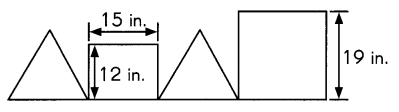The figure is made up of two identical triangles, a 15-inch by 12-inch rectangle and
a square of side 1 9 inches. What is the length of one side of each triangle if all the sides
of the triangles are equal in length?
15 inch is the length of one side of each triangle,

Explanation:
Rectangle = 15 + 12 + 15 +12 = 54 inches,
Square = 19 x 4 = 76 inches,
220 – ( 54 + 76) = 90 inches,
2 triangles = 6 sides = 90 ÷ 6 = 15 inch is the length of one side of each triangle.

Question 85.
A shop owner bought 260 handbags at 5 for $25. She then sold all of them at 2 for$1 8.
How much money did she make?
$1,040, Explanation: A shop owner bought 260 handbags at 5 for$25,
260 ÷ 5 = 52,
52 x 25 = 1300,
she bought the hand bag for $1300, She then sold all of them at 2 for$18.
260 ÷ 2 = 130,
130 x 18 = $2,340, She sold them for$2,340,
Total money she makes
$2,340 –$1,300 = $1,040. Solve. Show your work. Question 86. Alan scored a total of 14 points for answering all 15 questions on a math quiz. For every correctly answered question, Alan got 2 points. For every wrong answer, he lost 2 points. How many questions did he answer correctly? Answer: He answered 11 questions correctly, Explanation: For every correctly answered question, Alan got 2 points. For every wrong answer, he lost 2 points.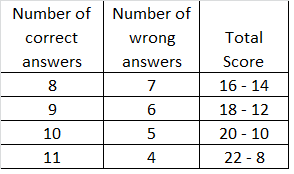Question 87. Beth and Lewis buy the same amount of fish flakes. If Beth feeds her goldfish 14 fish flakes each day, a container of flakes will last 20 days. If Lewis feeds his goldfish 8 fish flakes each day, how many more days will the container of flakes last for Lewis? Answer: The container will last 15 more days, Explanation: Beth feeds her goldfish 14 fish flakes each day, a container of flakes will last 20 days. 14 x 20 = 280, If Lewis feeds his goldfish 8 fish flakes each day. 280 ÷ 8 = 35, 35 days – 20 days = 15 days, The container will last 15 more days. Solve. Show your work. Question 88. Joan can pick 9 pounds of strawberries in one hour. a. How long does she take to pick 72 pounds of strawberries? Answer: 8 hours, Explanation: 72 pounds ÷ 9 pounds = 8, Joan takes 8 hours to pick 72 pounds. b. Joan is paid$12 per hour. How much does Joan earn if she picks twice the
total weight of strawberries in a.?
Joan earn $192, Explanation: 8 hrs x 2 = 16 hrs, 16 x$12 = $192, Joan earn$192.

Question 89.
There are 2,488 students in Washington School. There are 160 more students in Kent School.
The number of students in Bellow School is half the total number of students in
Washington School and Kent School. How many students are there in Bellow School?
There are 2,568 students in the Bellow school,

Explanation:
There are 2,488 students in Washington School.
There are 160 more students in Kent School.
2,488+ 160 = 2,648 students,
The number of students in Bellow School is half the total number of students in
Washington School and Kent School.
2,488 students + 2,648 students = 5,136 students,
Total students in Bellow School
5,136 students ÷ 2 = 2,568 students.